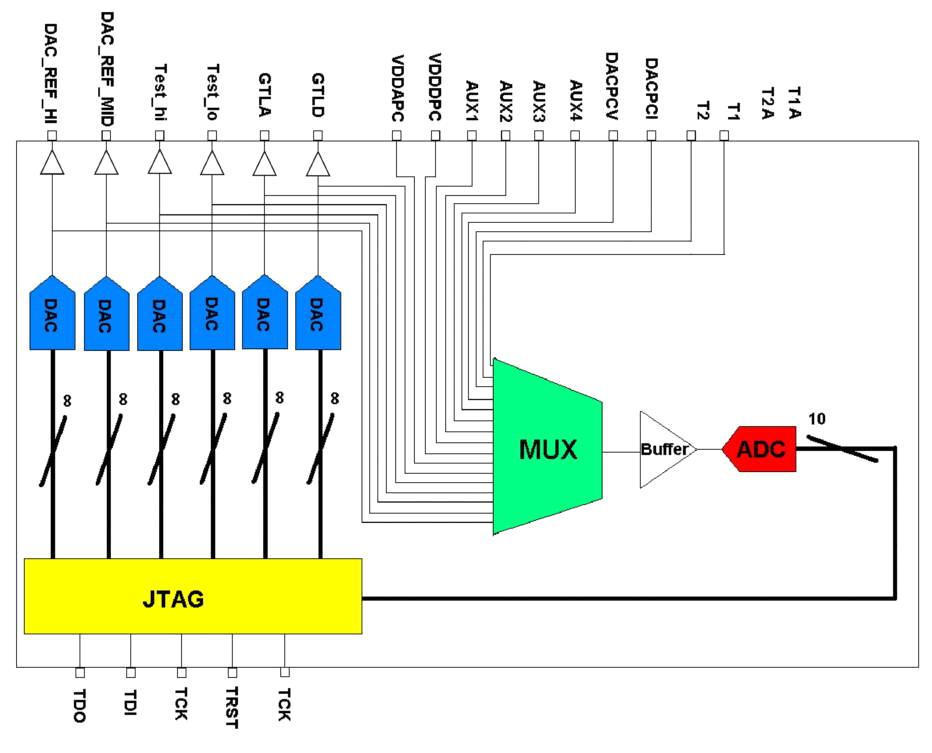# Analog Pilot conversion

Here is the description of the analog pilot conversion for the 16 ADC values shifted out by the jtag interface.

Below you see a diagram of the ADC connections in the anapil :There are two ways of calculating these values

### 1 - If you DON'T have the data collected during the half-stave and MCM tests

You can approximate the corresponding voltage measured by the ADC using the next formula:

Vmeasured = 1.379*adcCount + 513       [mV]


For the values read for the 6 DACs (DAC_REF_HI, DAC_REF_MID, TEST_HI, TEST_LOW, GTL_REF), a delta has to be applied due to a voltage drop in the grounding of the pixel bus. These deltas can be found in Bari's database or read from a configuration file for the corresponding half-stave .

It is known that the anapil current changes from half-stave to half-stave; from the values collected by Michele Caselle during the router interlock calibration, it was found out that a good guess is 0.245.

For calculating the BUS and MCM temperatures you can use the following formulas :

Rtbus = (1.379*adcCount + 513)/0.245       [ohm]
Tbus = (Rtbus-5000)/19.25                  [Cº]      -> pt5000 formula

Rmcm = (1.379*adcCount + 513)/0.245        [ohm]
Tmcm = B/( log(Rmcm/R0) + B/T0 ) - 273.15  [Cº]      -> NTC formula

Where R0 = 4700.00  [ohm]
T0 = 298.15   [K]
B = 3500.00


### 2 - If you have the data collected during the halfstave and mcm tests

If you have the data collected during the half-stave and MCM tests, then you will find the next parameters:

DeltaADCRefHi;
DeltaADCRefMid;
DeltaADCGtlRef;
DeltaADCTestHi;
DeltaADCTestLow;

Ipt
ADCSenseV;
ADCSenseI2;
ADCPixelVdd;

The first 5 values are the deltas to apply for the measurements of the anapil DACS.

Ipt is the measure of the current injected to measure the temperatures.

ADCSenseV is an array mapping the voltage measured by the adc corresponding to a certain adc count.

ADCSenseI2 is an array mapping the measurement of <nop>SenseI.

ADCPixelVdd is the mapping of the <nop>VddPilot voltage to a certain adc count.

so :

Vmeasure = ADCSenseV[adcCount]      [mV]
SenseI = ADCSenseI2[adcCount]
VddPilot = 2*ADCPixelVdd[adcCount]  [mV]

Rtbus = ADCSenseV[adcCount]/Ipt (then use pt5000 formula to get the temperature, see above)
Rtmcm = ADCSenseV[adcCount]/Ipt (then use NTC formula to get the the temperature, see above)


All other values just use the corresponding voltage measured by the adc (<nop>Vmeasured = <nop>ADCSenseV[adcCount])

Calculation_of_temperatures_and_statistical_distribution_read_from_Router_for_monitoring_and_interlock (Calculation of the temperatures doc, thanks to Michele)

SPD_temperature_calibration_final_statistical (router temperature calibration excel sheet)